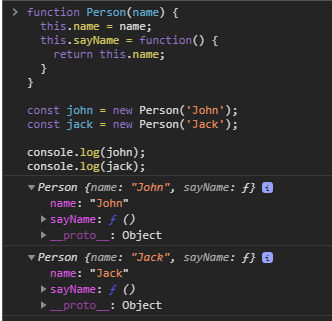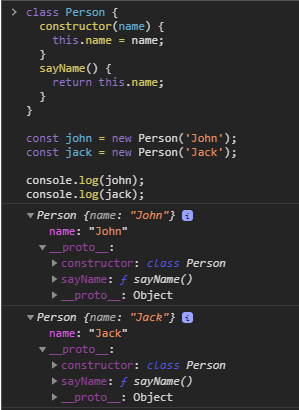# What kind of a difference does a prototype make?

Hello,
I am still learning OOP and I don’t understand the role of prototypes.
Why is it better to write this:

``````function Object1{
this.x = 1;
this.y = 2;
}
Object1.prototype.multiply = function(){console.log(x+y)}
``````

``````function Object1{
this.x = 1;
this.y = 2;
this.multiply = function(){console.log(x+y)}
}
``````

The challenge on prototypes does explain it, does it not?

1 Like

I went through that challenge and watched some videos on YouTube and I still don’t understand what kind of difference does it make. I both cases the method is called the same way.
I understand that I am supposed to make a prototype when a property is shared between more instances of an object but I don’t understand why would I do that and write extra code when I can pack that property right into the object.

Because then it’s only on that object. Say you have a type of Object1 (ie one that inherits from Object1). It’s not going to have that. As an example, do something like

``````> o1 = new Object1();
> o1.toString()
> o1.valueOf()
``````

You didn’t add those methods, yet they are there just the same.

1 Like

It’s already been said, but I’m just pointing it out again for clarity.

If you do this:

``````function Object1{
this.x = 1;
this.y = 2;
this.multiply = function(){console.log(x+y)}
}
``````

and create 1000 Objects, they will all have their own multiply function. That’s 1000 variables in memory.

If you do this:

``````function Object1{
this.x = 1;
this.y = 2;
}
Object1.prototype.multiply = function(){console.log(x+y)}
``````

and create 1000 Objects, there will only be one multiply function in memory.

For small pieces of code, this doesn’t matter much. But imagine a social media website with millions of users.

1 Like

@jsdisco I think you missed your parentheses.

As said, it’s just a way of making all instances share the same values through the prototype (like methods, or other data).

Without proto:With proto:Methods by default when using the `class` syntax are on the prototype (`__proto__` property).

Class Methods:I just copied the two code examples from the OP, I didn’t even realise that the multiply function is actually an add function… but I’m too blind to find the missing parentheses?

Ah, I didn’t notice that.

I mean, I assume it’s supposed to be a constructor function?

``````function Object1() {
this.x = 1;
this.y = 2;
}
``````

Edit: Just to be super clear about it and seeing as it’s the OPs code.

Original code without parentheses (invalid syntax):

``````function Object1{
this.x = 1;
this.y = 2;
}
Object1.prototype.multiply = function(){console.log(x+y)}

function Object1{
this.x = 1;
this.y = 2;
this.multiply = function(){console.log(x+y)}
}
``````

With parentheses:

``````function Object1() {
this.x = 1;
this.y = 2;
}
Object1.prototype.multiply = function(){console.log(x+y)}

function Object1() {
this.x = 1;
this.y = 2;
this.multiply = function(){console.log(x+y)}
}
``````

Must be side effects, I’ve been staring at object literals a lot today.